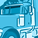QlikView App Dev

Discussion Board for collaboration related to QlikView App Development.

Announcements
QlikWorld 2022, LIVE in Denver CO., May 16-19, 2022. REGISTER NOW TO RECEIVE EARLY BIRD PRICING
cancel
Showing results for
Did you mean:Contributor II

Greater than average of top 5 values

I have a straight table in the form:

 Name Value a 3 b 6 c 2 d 7 e 4 f 1 g 2 h 8 i 4 j 3 k 5 l 1 m 5 n 3

I need some help with being able to highlight those values which are greater than average of top 5 values.

In this case, top five values are: 8,7,6,5,5. and average of these values would be 6.2. So all the values in the table >6.2 need to be highlighted in a colour say blue.

I have tried using:

if(Values > if(rank(Values)<=5, sum(Values))/5, LightBlue(),LightGray())
in the 'Background Colour' option of the Values expression but it doesn't seem to work. Can this be done in the front end using the table options itself?

Labels (1)
• Layout & Visualizations

1 Solution

Accepted SolutionsMVP

HI @nora7

Try like below

Color expression: If(Sum(Value) >= vRankAvgValue, LightBlue(),LightGray())

vRankAvgValue

=Avg(Aggr(If(Rank(Sum(Value),4) <= 5, Sum(Value)), Name))Thanks & Regards,
Mayil Vahanan R
3 RepliesPartner

Maybe this is a solution.

In background expression:

=if(
isnull(
avg({\$<Name={"=num(subfield(rank(Value,1,3),'-',-1))<=5"}>}Value)
)
,LightGray()
,LightBlue()
)MVP

HI @nora7

Try like below

Color expression: If(Sum(Value) >= vRankAvgValue, LightBlue(),LightGray())

vRankAvgValue

=Avg(Aggr(If(Rank(Sum(Value),4) <= 5, Sum(Value)), Name))Thanks & Regards,
Mayil Vahanan RContributor II
Author

Thank you so much @eddie_wagt and @MayilVahanan , this really helps!Community Browser